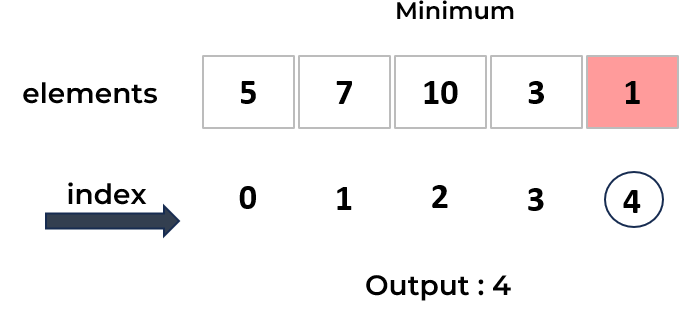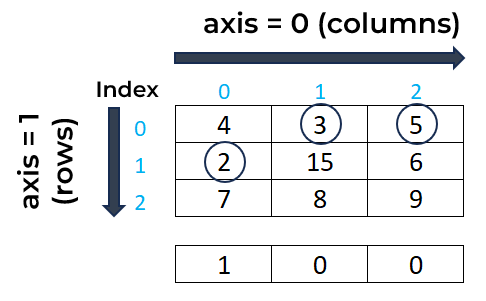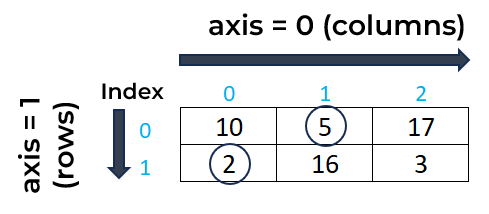# NumPy argmin() Function : Learn with Examples

In this tutorial, we will see how to use the NumPy argmin() function in Python along with examples.The numpy.argmin() function in Python is used to find the indices of the minimum element in an array.

## Syntax of NumPy argmin() Function

Below is the syntax of the NumPy argmin() function:

```import numpy as np
np.argmin(array, axis, out)
```
• array : NumPy array.
• axis : (optional). Specify the axis to find the indices of the minimum value in two dimensional arrays.
• out : (optional). It is used to store the output of the function.
Example 1 : argmin() Function in One-Dimensional Array

The following code uses the argmin() function from the NumPy package to return the index of the smallest value in the one-dimensional array named "my_array".In this array, the minimum value is 1 which lies at the 4th index. Keep in mind that indexing starts from 0 in python so the 4th index refers to the fifth element in the array.

```import numpy as np
my_array = np.array([5,7,10,3,1])
np.argmin(my_array)
```
``````
# Output
4
```
```
Example 2 : argmin() Function in Two-Dimensional Array

In this example, we have created a sample 2D array. In this array, the smallest value is 2 which lies at the 3rd index as indexing starts from 0 in python instead of 1.

```# 2D array
my_array = np.array([[4, 3, 5], [2, 15, 6], [7, 8, 9]])

# Index of the minimum element
np.argmin(my_array)
```
``````
# Output
3
```
```
Example 3 : Finding Minimum Element Indices per Column

When we use `axis=0` argument in the argmin() function, it means that we want to find the indices of the minimum elements along each column of the array. In simple words, it returns the index of the minimum value for each column.```# 2D array
my_array = np.array([[4, 3, 5], [2, 15, 6], [7, 8, 9]])

# Finding the indices of the minimum elements along each column (axis=0)
print(np.argmin(my_array, axis=0))
```
``````
# Output
[1 0 0]
```
```
Example 4 : Finding Minimum Element Indices per Row

When we use `axis=1` argument in the argmin() function, it means that we want to find the indices of the minimum elements along each row of the array. In simple words, it returns the index of the minimum value for each row.```# 2D array
my_array = np.array([[10,5,17],[2,16,3]])

# Finding the indices of the minimum elements along each row (axis=1)
print("Indices of minimum elements per row:", np.argmin(my_array, axis=1))
print("Indices of minimum elements per column:", np.argmin(my_array, axis=0))
```
``````
# Output
Indices of minimum elements per row: [1 0]
Indices of minimum elements per column: [1 0 1]
```
```
Example 5 : Store Result using out Parameter

We can use the `out` parameter in the argmin() function to store the result which is the index of the minimum element.

```my_array = np.array([5,7,10,3,1])

# Creating an output array to store the index of minimum element
out_array = np.array(0)
np.argmin(my_array,out=out_array)
print("Index of minimum element:",out_array)
```
``````
# Output
Index of minimum element: 4
```
```

In two-dimensional array, it is important to create an empty NumPy array with the number of columns in "my_array" and integer data type to store result.

```my_array = np.array([[7, 4, 6], [6, 5, 7], [7, 8, 9]])

# Creating an output array to store the indices of minimum elements along each column
out_array = np.empty(my_array.shape, dtype=int)

np.argmin(my_array, axis=0, out=out_array)
print("Indices of minimum elements:",out_array)
```
``````
# Output
Indices of minimum elements: [1 0 0]
```
```
Related Posts